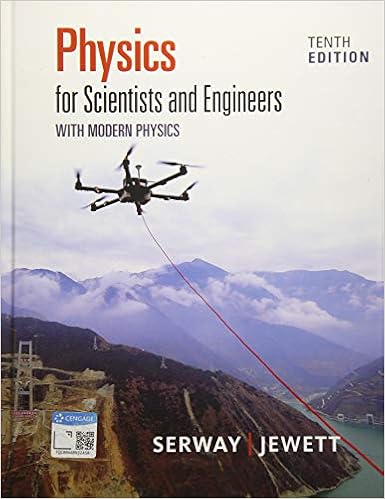# Both wires above are in parallel with equal currents

• 19
• 100% (3) 3 out of 3 people found this document helpful

This preview shows page 11 - 15 out of 19 pages.

##### We have textbook solutions for you!
The document you are viewing contains questions related to this textbook.The document you are viewing contains questions related to this textbook.
Chapter 23 / Exercise 02
Physics for Scientists and Engineers with Modern Physics
Jewett/SerwayExpert Verified
29.Both wires above are in parallel with equal currents flowing in opposite directions. At what location would the magnetic fields cancel out?a.Anywhere on the line xx’b.Anywhere on the line yy’c.Anywhere on the line Ax’d.At no points on the diagram
##### We have textbook solutions for you!
The document you are viewing contains questions related to this textbook.The document you are viewing contains questions related to this textbook.
Chapter 23 / Exercise 02
Physics for Scientists and Engineers with Modern Physics
Jewett/SerwayExpert Verified
Questions 30-31A magnetic field of 1 T causes a proton beam of 2 mA to move in a circle with a radius of 0.1 m. Assume that the magnetic field is perpendicular to the plane that the circle is located on.30.What is the best estimate of the work done in Joules on the protons in each orbit of the complete circle?
31.What is the best estimate of the speed of a single proton in this beam of protons moving in a circle due to the magnetic field?
32.Shown above there is an electron moving to the right undeflected by both the electric field in between the plates and the magnetic field coming out of the page in between the plates. The magnetic field has a magnitude of 2.0 T and the electric field has a magnitude of 8 N/C. What is the speed of the electron?
33.A particle with a negative charge has an initial velocity that is parallel to a magnetic field moving to the right. What path would this negatively charged particle follow?a.Parabolic arcb.Spiralc.Circular arcd.Straight line parallel to the field
•••